# Determinant facts for kids

Kids Encyclopedia Facts

The determinant of a square matrix is a scalar (a number) that indicates how that matrix behaves. It can be calculated from the numbers in the matrix.

The determinant of the matrix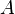$A$ is written as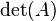$\det(A)$ or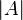$|A|$ in a formula. Sometimes, instead of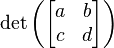$\det\left(\begin{bmatrix}a&b\\c&d\end{bmatrix}\right)$ and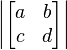$\left|\begin{bmatrix}a&b\\c&d\end{bmatrix}\right|$, one simply writes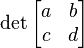$\det\begin{bmatrix}a&b\\c&d\end{bmatrix}$ and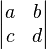$\left|\begin{matrix}a&b\\c&d\end{matrix}\right|$.

## Singular matrices

A matrix has an inverse matrix exactly when the determinant is not 0. For this reason, a matrix with a non-zero determinant is called invertible. If the determinant is 0, then the matrix is called non-invertible or singular.

Geometrically, one can think of a singular matrix as "flattening" the parallelepiped into a parallelogram, or a parallelogram into a line. Then the volume or area is 0, which means that there is no linear map that will bring the old shape back.

## Calculating a determinant

There are a few ways to calculate a determinant.

### Formulas for small matrices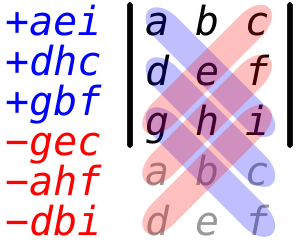The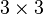$3 \times 3$ determinant formula is a sum of products. Those products go along diagonals that "wrap around" to the top of the matrix. This trick is called the Rule of Sarrus.
• For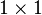$1 \times 1$ and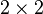$2 \times 2$ matrices, the following simple formulas hold: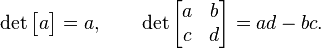$\det\begin{bmatrix}a\end{bmatrix} = a,\qquad\det\begin{bmatrix}a&b\\c&d\end{bmatrix} = ad-bc.$

• For$3 \times 3$ matrices, the formula is:${\det\begin{bmatrix}a&b&c\\d&e&f\\g&h&i\end{bmatrix} = {\color{blue}{aei}+{dhc}+{gbf}}{\color{OrangeRed}{}-{gec}-{ahf}-{dbi}}}$

One can use the Rule of Sarrus (see image) to remember this formula.

### Cofactor expansion

For larger matrices, the determinant is harder to calculate. One way to do it is called cofactor expansion.

Suppose that we have an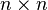$n \times n$ matrix$A$. First, we choose any row or column of the matrix. For each number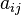$a_{ij}$ in that row or column, we calculate something called its cofactor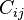$C_{ij}$. Then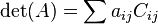$\det(A) = \sum a_{ij} C_{ij}$.

To compute such a cofactor$C_{ij}$, we erase row$i$ and column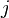$j$ from the matrix$A$. This gives us a smaller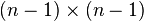$(n-1)\times(n-1)$ matrix. We call it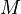$M$. The cofactor$C_{ij}$ then equals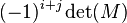$(-1)^{i+j} \det(M)$.

Here is an example of a cofactor expansion of the left column of a$3 \times 3$ matrix: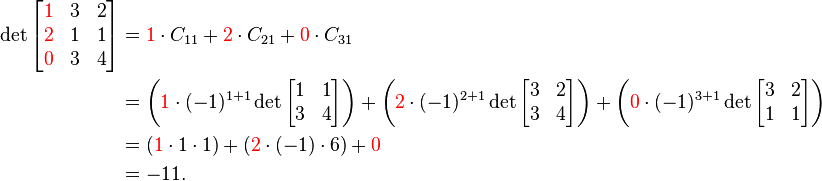\begin{align} \det \begin{bmatrix}{\color{red}1}&3&2\\{\color{red}2}&1&1\\{\color{red}0}&3&4\end{bmatrix} &= {\color{red}1} \cdot C_{11} + {\color{red}2} \cdot C_{21} + {\color{red}0} \cdot C_{31} \\ &= \left( {\color{red}1} \cdot (-1)^{1+1} \det\begin{bmatrix}1&1\\3&4\end{bmatrix} \right) + \left( {\color{red}2} \cdot (-1)^{2+1} \det\begin{bmatrix}3&2\\3&4\end{bmatrix} \right) + \left( {\color{red}0} \cdot (-1)^{3+1} \det\begin{bmatrix}3&2\\1&1\end{bmatrix} \right) \\ &= ({\color{red}1} \cdot 1 \cdot 1) + ({\color{red}2} \cdot (-1) \cdot 6) + {\color{red}0} \\ &= -11. \end{align}

As illustrated above, one can simplify the computation of determinant by choosing a row or column that has many zeros; if$a_{ij}$ is 0, then one can skip calculating$C_{ij}$ altogether.

## Related pagesDeterminant Facts for Kids. Kiddle Encyclopedia.